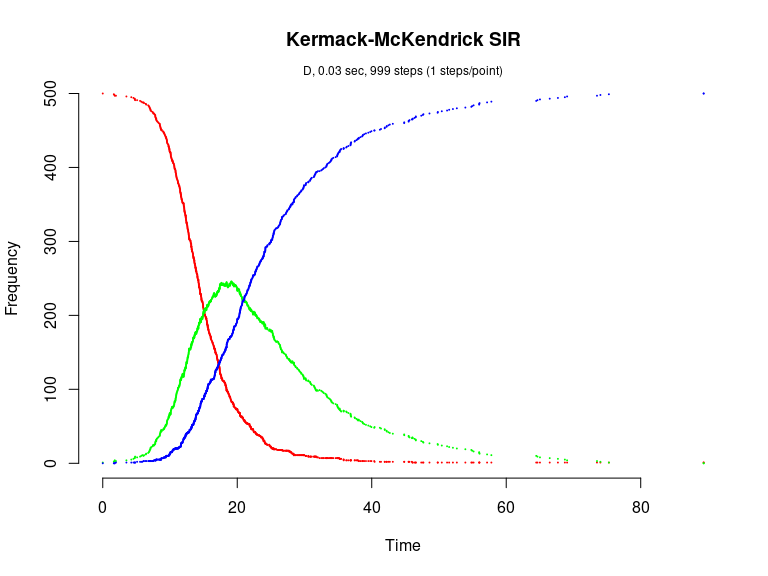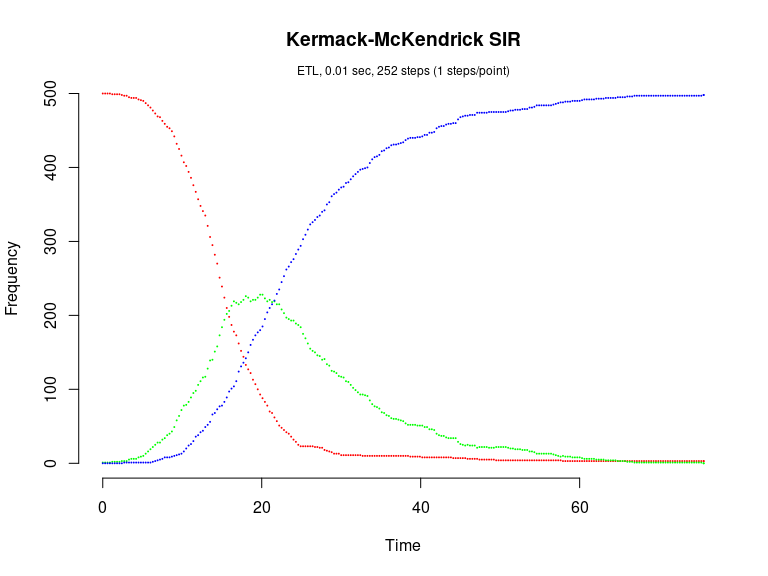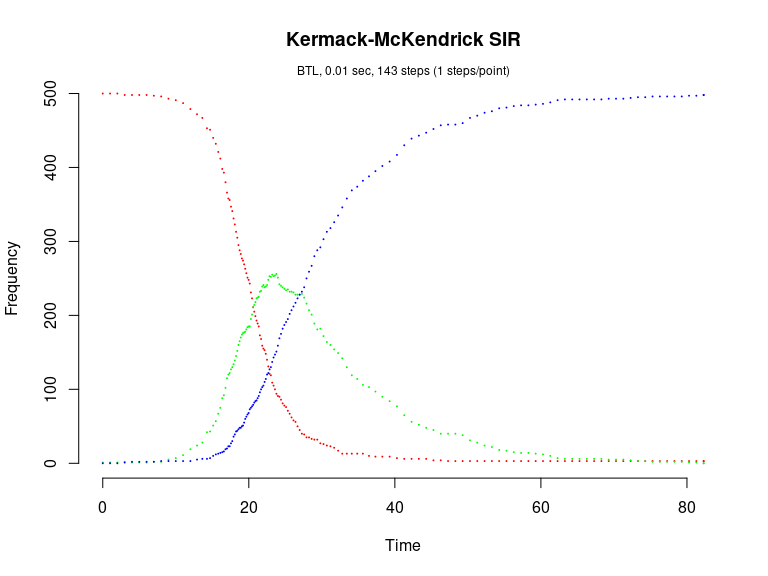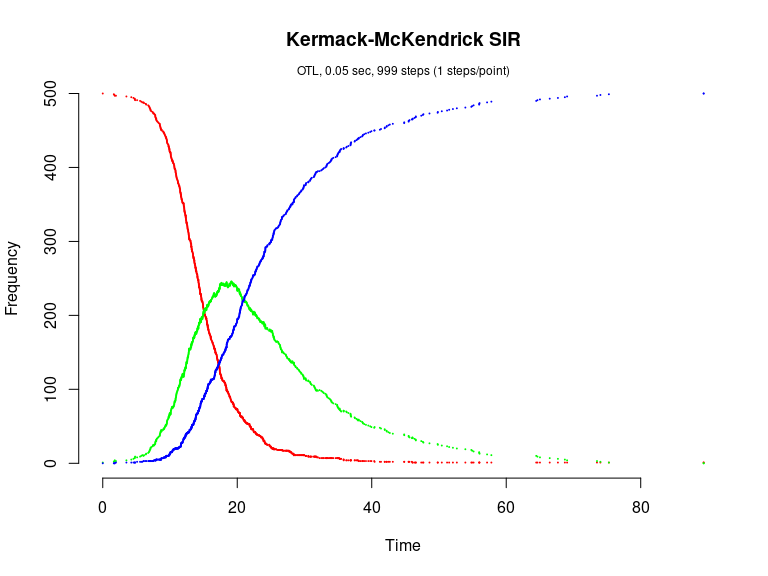# Kermack-McKendrick SIR model (Brown & Rothery, 1993)

The Kermack-McKendrick SIR model is defined as

dS/dt = -beta*N*S
dI/dt = beta*N*S - gamma*I
dR/dt = gamma*I

This model consists of two reactions with the following per capita rates,

transmission: beta
recovery:     gamma

library(GillespieSSA)

Define parameters

parms <- c(beta=.001, gamma=.100)
tf <- 100                                      # Final time
simName <- "Kermack-McKendrick SIR"            # Name

Define initial state vector

x0 <- c(S=500, I=1, R=0)

Define state-change matrix

nu <- matrix(c(-1,0,1,-1,0,1),nrow=3,byrow=TRUE)

Define propensity functions

a  <- c("beta*S*I", "gamma*I")

Run simulations with the Direct method

set.seed(1)
out <- ssa(
x0 = x0,
a = a,
nu = nu,
parms = parms,
tf = tf,
method = ssa.d(),
simName = simName,
verbose = FALSE,
consoleInterval = 1
)
ssa.plot(out, show.title = TRUE, show.legend = FALSE)Run simulations with the Explict tau-leap method

set.seed(1)
out <- ssa(
x0 = x0,
a = a,
nu = nu,
parms = parms,
tf = tf,
method = ssa.etl(),
simName = simName,
verbose = FALSE,
consoleInterval = 1
)
ssa.plot(out, show.title = TRUE, show.legend = FALSE)Run simulations with the Binomial tau-leap method

set.seed(2) # for some reason, this does not work with seed = 1
out <- ssa(
x0 = x0,
a = a,
nu = nu,
parms = parms,
tf = tf,
method = ssa.btl(),
simName = simName,
verbose = FALSE,
consoleInterval = 1
)
ssa.plot(out, show.title = TRUE, show.legend = FALSE)Run simulations with the Optimized tau-leap method

set.seed(1)
out <- ssa(
x0 = x0,
a = a,
nu = nu,
parms = parms,
tf = tf,
method = ssa.otl(),
simName = simName,
verbose = FALSE,
consoleInterval = 1
)
ssa.plot(out, show.title = TRUE, show.legend = FALSE)